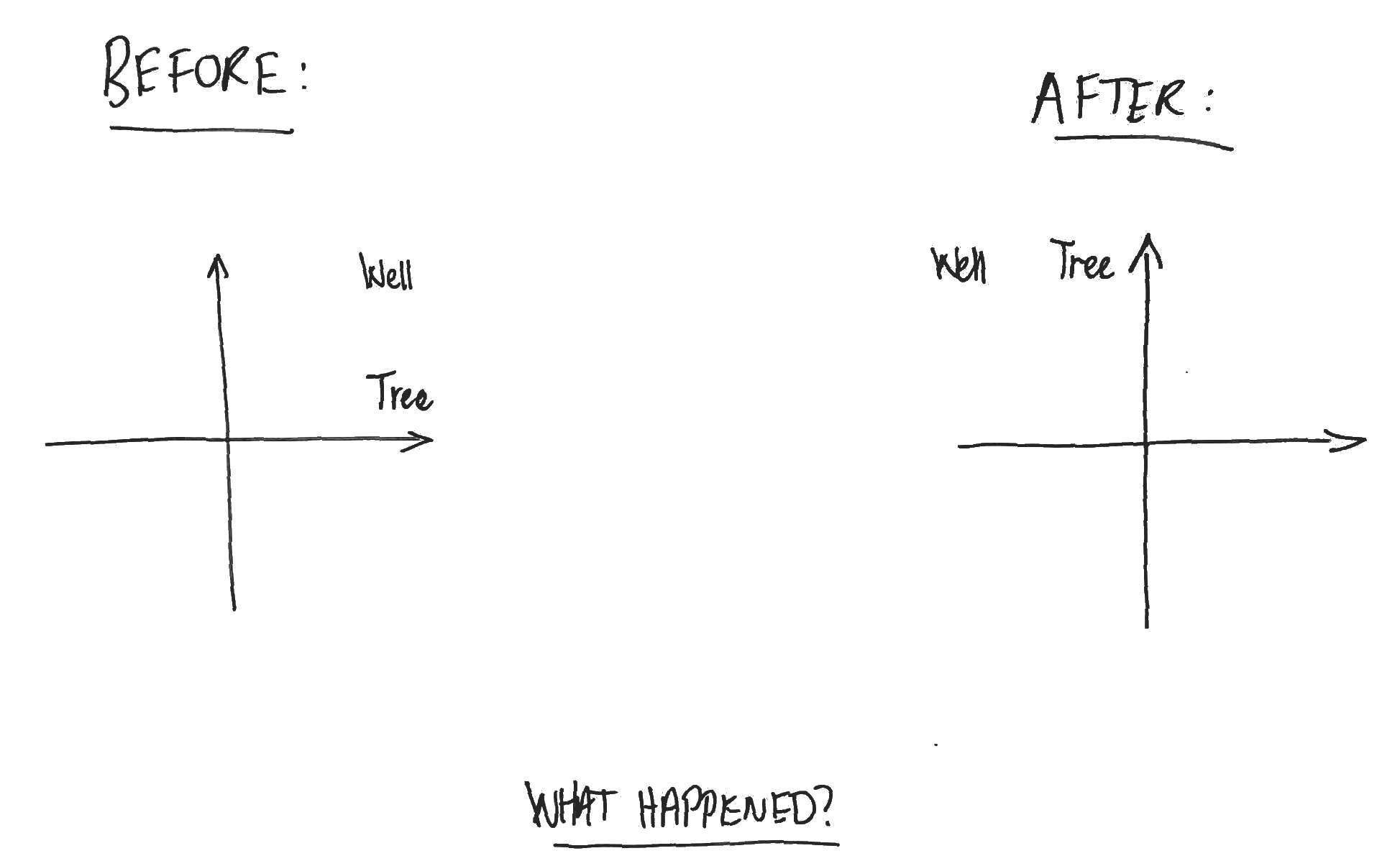## Descartes’ headache: rotation of space is transpose to rotation of co-ordinate system

[I often speak of such-and-such depending upon the choice of co-ordinate system, and proceed to show this by rotating the space. The following explains why this is equivalent (via the transpose)].

You awaken in a two-dimensional landscape. In front of you are co-ordinate axes, fixed to the ground on a pivot. The landscape is featureless except for a tree and a well. You get bored, and fall asleep again. When you awaken, you notice that the relative arrangement of the co-ordinate axes to the tree and the well is different. What happened?You realise that you’ll never truly know what happened while you were asleep. Either (a) someone rotated the co-ordinate axes 90 degrees clockwise, or (b) someone rotated the world 90 degrees anti-clockwise about the pivot point of the axes.

Rotation of the space is equivalent (via the transpose) to rotation of the co-ordinate system. In fact, this is true more generally for orthogonal transformations and has a familiar mathematical expression. Let $V$ be an n-dimensional vector space with inner product $\langle \cdot , \cdot \rangle$ and let $e_i, i=1, .., n$ be an orthonormal basis. If $X$ is an orthogonal transformation of $V$, then for any point $v \in V$, we have
$$\displaystyle \langle Xv, e_i \rangle = \langle v, X^T e_i \rangle$$

for all $i=1, .. , n$. That is, the co-ordinates of the transformed point with respect to the original basis are the co-ordinates of the original point with respect to the transpose-transformed basis.